Next: Multi-Variate Gaussian Intensity Prior Up: Flat Intensity Prior Previous: Marginalising over Areas of

### Pure Partial Volume Parameters

Some parameters are associated only with a small partial volume effect, normally at a single voxel, which can occur when a shape of interest or the area of no interest partially overlaps a measured voxel. The parameters will be called pure partial volume parameters,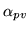here. In this case the associated column of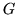has a norm less than 1.0, and the finite range of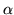can no longer be ignored. Hence the approximation of using equation 13 (in appendix A) must be replaced by the more accurate integral of equation 14. However, in this case the region of integration also becomes important. In the preceding sections the variables are often transformed in order to compute the integrals (the substitution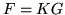which is done in appendix A).

For typical models, most columns of, apart from those associated with, have norms that are much larger than 1.0, and are almost orthogonal with the columns associated with these partial volume parameters. Consequently, the effect of the change of variables is to induce a slight rotation in the effective region of integration. However, this effect is small and will be ignored here.

Applying the previous marginalisations of otherparameters first, leads to a posterior of the form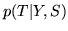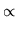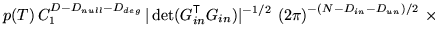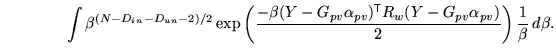Using equation 14 and assuming that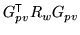has negligible off-diagonal terms gives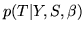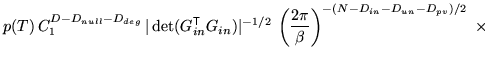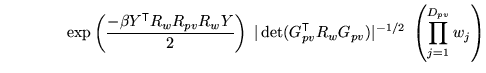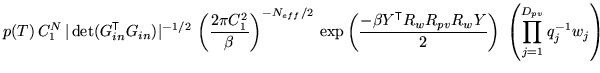(9)

where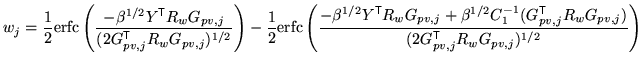represents a general weighting factor for the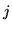th voxel (associated with theth partial volume parameter);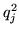is theth diagonal element of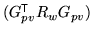which represents the squared partial volume fraction of theth partial volume parameter;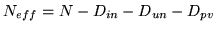are the effective number of degrees of freedom for this model; and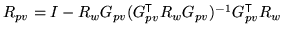is the residual projection matrix including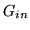,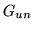and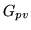. The projection matrix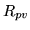can also be calculated more straightforwardly from the pseudo-inverse ofas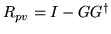, where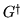is the pseudo-inverse of.

Note that it is now no longer possible to easily marginalise over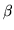as it appears in the complimentary error functions. Consequently, the posterior has been left in the formwhich can either be used with a pre-specified value of(e.g. by estimating the SNR from the image) or numerically marginalised.

Using the fact that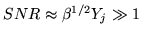and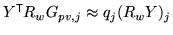gives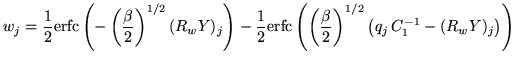which is a monotonic function of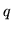.

Furthermore, as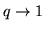then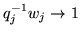, and as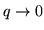then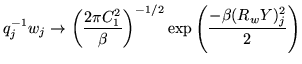The consequence of this is that when the partial volume overlap becomes large () the posterior assumes the form that would result if this parameter was part of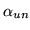, that is a voxel wholly inside the area of no interest. This is what would occur as the transformation moved in such a way as to move this measured voxel wholly into the area of no interest. Therefore the posterior is continuous with respect to this change in transformation.

Also, as the partial volume overlap becomes small () then the posterior assumes the form that would result by increasing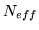by 1 and including the residual intensity mismatch from voxelback into the general residual term. This is what would happen as this voxel makes the transition fully into the measured area of interest, resulting in an extra effective voxel in the measurement (the increase in) and the full inclusion of the residual intensity error.

In between these extreme values the contribution becomes partial. This can be compared to the form of de-weighting used for voxels at the edge of the valid field of view, in , which can be expressed in this notation as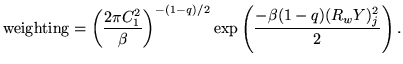This takes the same extreme values and is also monotonic, and thus performs the same function, albeit with a slightly different rate of change.

Note that the careful use of the finite range ofand the introduction of the complimentary error functions in the integrations is crucial for making the posterior a continuous function of the spatial transformation.Next: Multi-Variate Gaussian Intensity Prior Up: Flat Intensity Prior Previous: Marginalising over Areas of